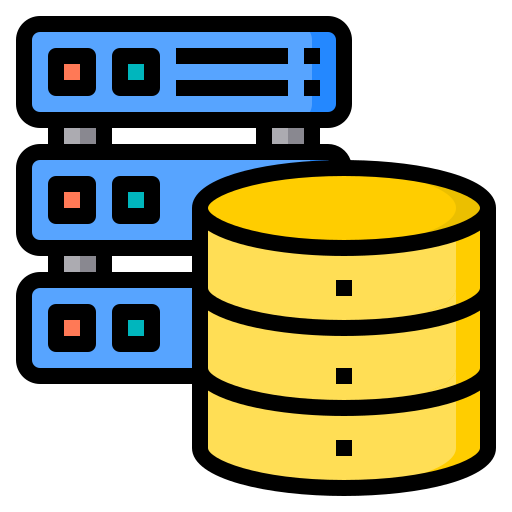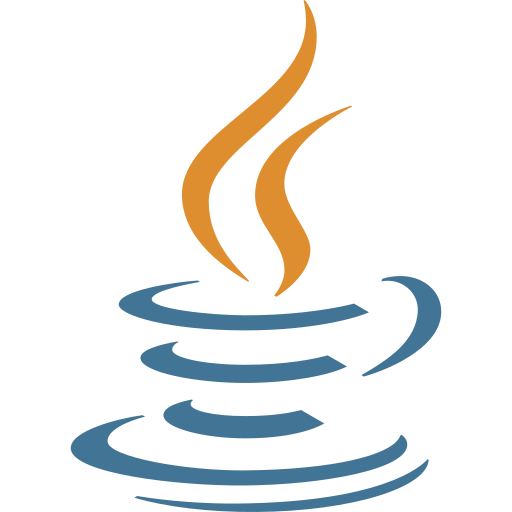Course Content

Java Basics

##Char and ASCII Table (bonus)## Why Can We Perform Mathematical Operations on the char Data Type?

The `char` data type is quite interesting. It stores characters, but you can also perform operations on this data type.

What will be the result of executing this code?Main.javaNote

Here, we use the "+" sign to concatenate two different variables. We will cover basic operations in the next chapter, but for now, you should know that "+" combines the values of two variables.

One might have thought that the result would be "`A(`", but as we can see, the result is "`i`". Why is that? `char` is not purely a character data type; it takes characters from the ASCII table. The character "`A`" corresponds to the number 65 in the table, while the character "`(`" corresponds to the number 40. As you may have guessed, the character "`i`" corresponds to 105, as 65 + 40 = 105. It's important to know this because it allows us to operate on the `char` data type excellently and flexibly.

Here is a link to the ASCII table.

Note:

Type casting in Java refers to the process of converting one data type to another. It allows us to treat a variable of one type as if it were another. When casting an `int` to a `char`, we can use the `(char)` syntax. This conversion is known as a narrowing primitive conversion because we convert from a larger range (32-bit integer) to a smaller range (16-bit Unicode character).

####Why can we perform mathematical operations on char?Everything was clear?

Section 2. Chapter 3